In a previous post I started trying to skeletonize a polygon to provide a nice centre line in an irregular polygon to provide a place for a label to be drawn.

I had worked out the skeletonization but was stuck on how to remove the “dangling” edges from the centre line that I actually wanted. The answer turned up in a couple of places at the same time - Julien on gis.stackexchange proposed it at roughly the same time that Jeff McKenna pointed Steve Lime (and the rest of the MapServer community) to Noah Veltman’s approach to the problem. This is to loop through all the “dangling” nodes (i.e. of index 1) and calculate the shortest distance to all the other dangling nodes and keep the longest one. Luckily for me GeoTools already has a graph module (`gt-graph`) which can do all of that for me.

``````private static Geometry reduceToCentreLine(Geometry geom) {
LineStringGraphGenerator gen = new LineStringGraphGenerator();
for (int i = 0; i < geom.getNumGeometries(); i++) {
}
Graph graph = gen.getGraph();
EdgeWeighter weighter = e -> {
Geometry g = (Geometry) e.getObject();
return g.getLength();
};
double bestLen = Double.NEGATIVE_INFINITY;
Path bestPath = null;
for (Node source : graph.getNodesOfDegree(1)) {
// calculate the cost(distance) of each graph node to the node closest to
// the origin
// System.out.println("starting at " + source.getObject());
DijkstraShortestPathFinder dspf = new DijkstraShortestPathFinder(graph, source, weighter);
dspf.calculate();
for (Node dest : graph.getNodesOfDegree(1)) {
// System.out.println("\troute to " + dest.getObject());
if (dest.equals(source)) {
continue;
}
// get length
double len = 0.0;
Path path = dspf.getPath(dest);
if (path == null) {// no path to dest
continue;
}
for (Edge e : path.getEdges()) {
Geometry g = (Geometry) e.getObject();
len += g.getLength();
}
if (len > bestLen) {
bestPath = path;
bestLen = len;
}
}
}
ArrayList<LineString> edges = new ArrayList<>();
for (Edge e : bestPath.getEdges()) {
Geometry g = (Geometry) e.getObject();
}
return GF.createMultiLineString(GeometryFactory.toLineStringArray(edges));
}
``````

So now I get this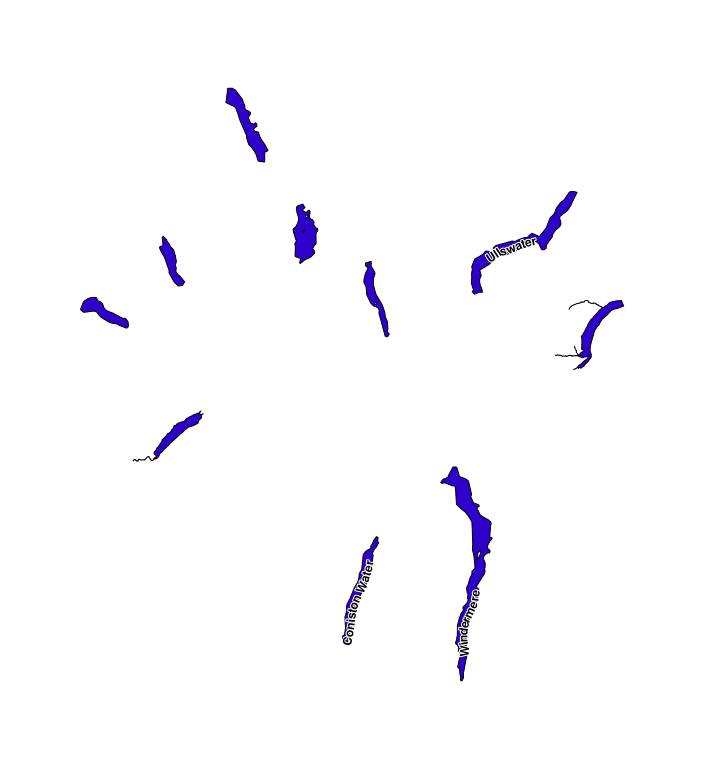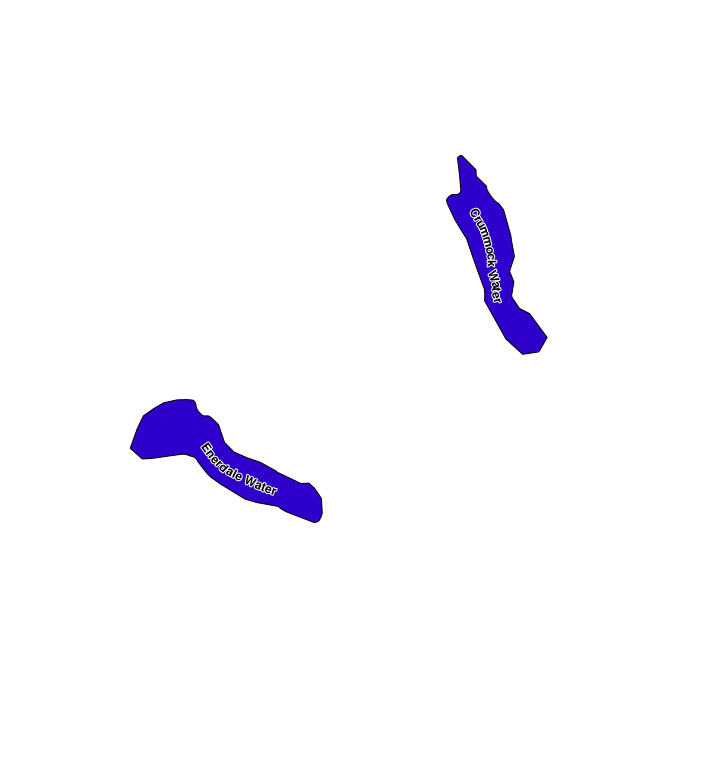which is starting to look good, it’s less good if you use it on more regular shapes like the US States.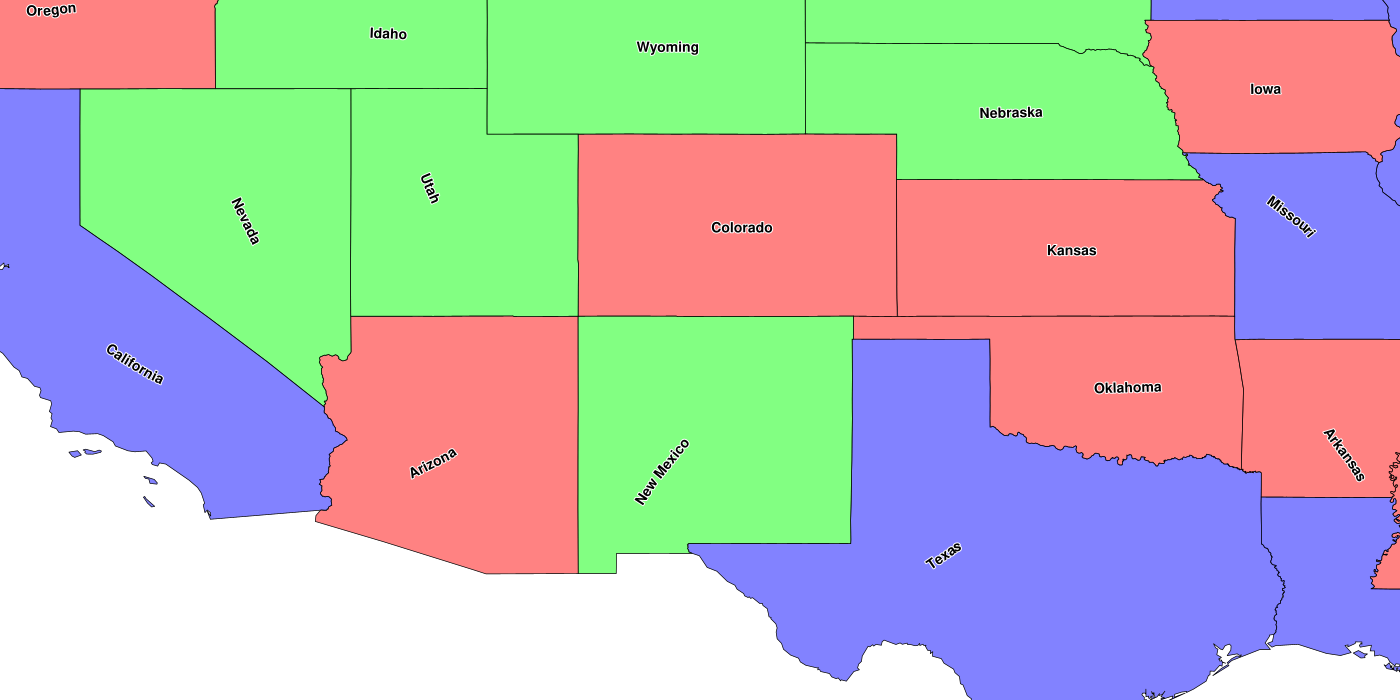As you can see there probably needs to be an optional check to see if the label will fit horizontally before moving to the centre line (e.g. New Mexico, Utah).

Currently, there is a magic tolerance variable that is used to control a simplify/densify step that makes sure that there are not too many points, which is slow or too spaced out which leads to breaks in the centre line.

``````    double dist = poly.getLength() * (perc_tolerance / 100);
Polygon spoly = (Polygon) TopologyPreservingSimplifier.simplify(poly, dist / 100.0);
Polygon vpoly = (Polygon) Densifier.densify(spoly, dist);
``````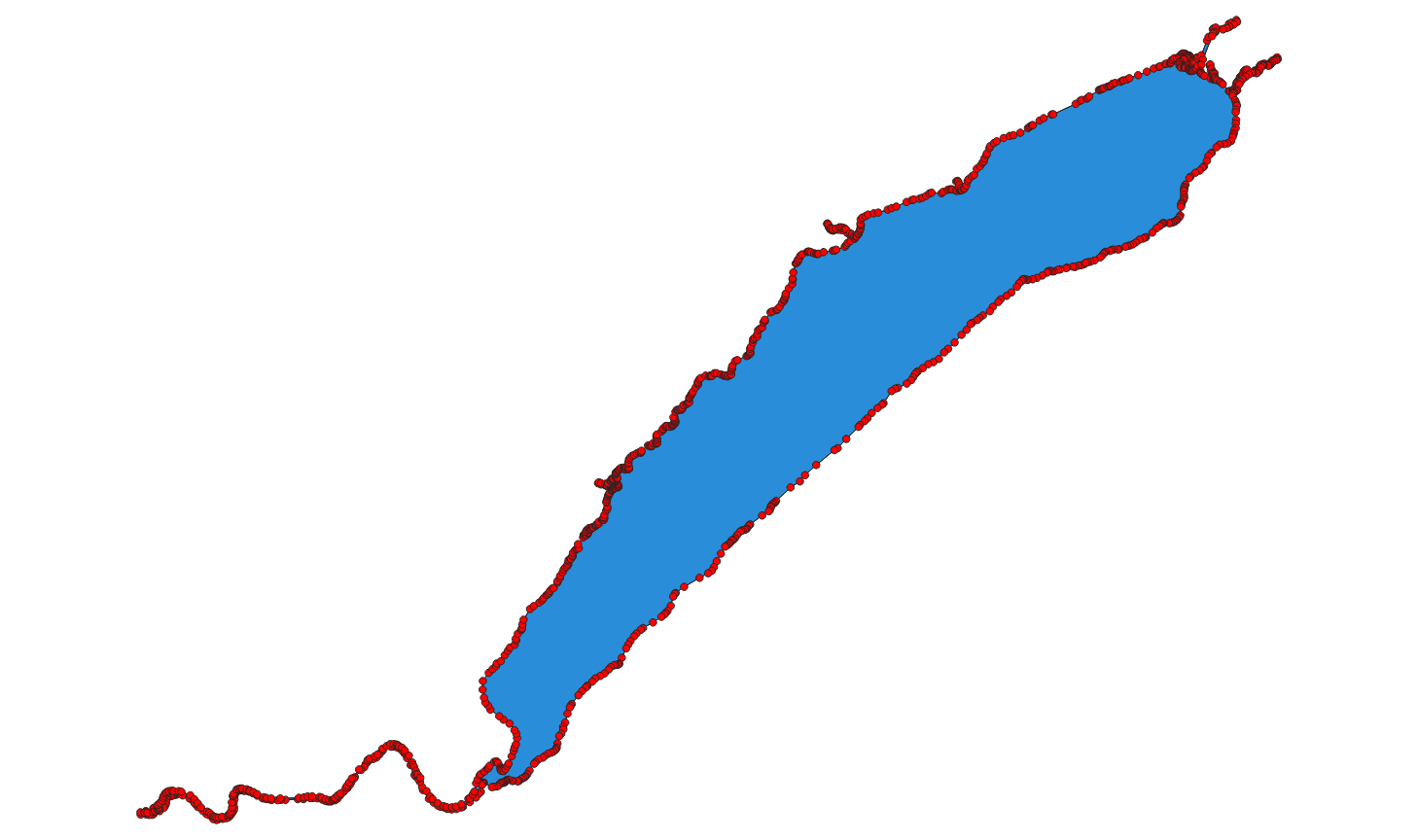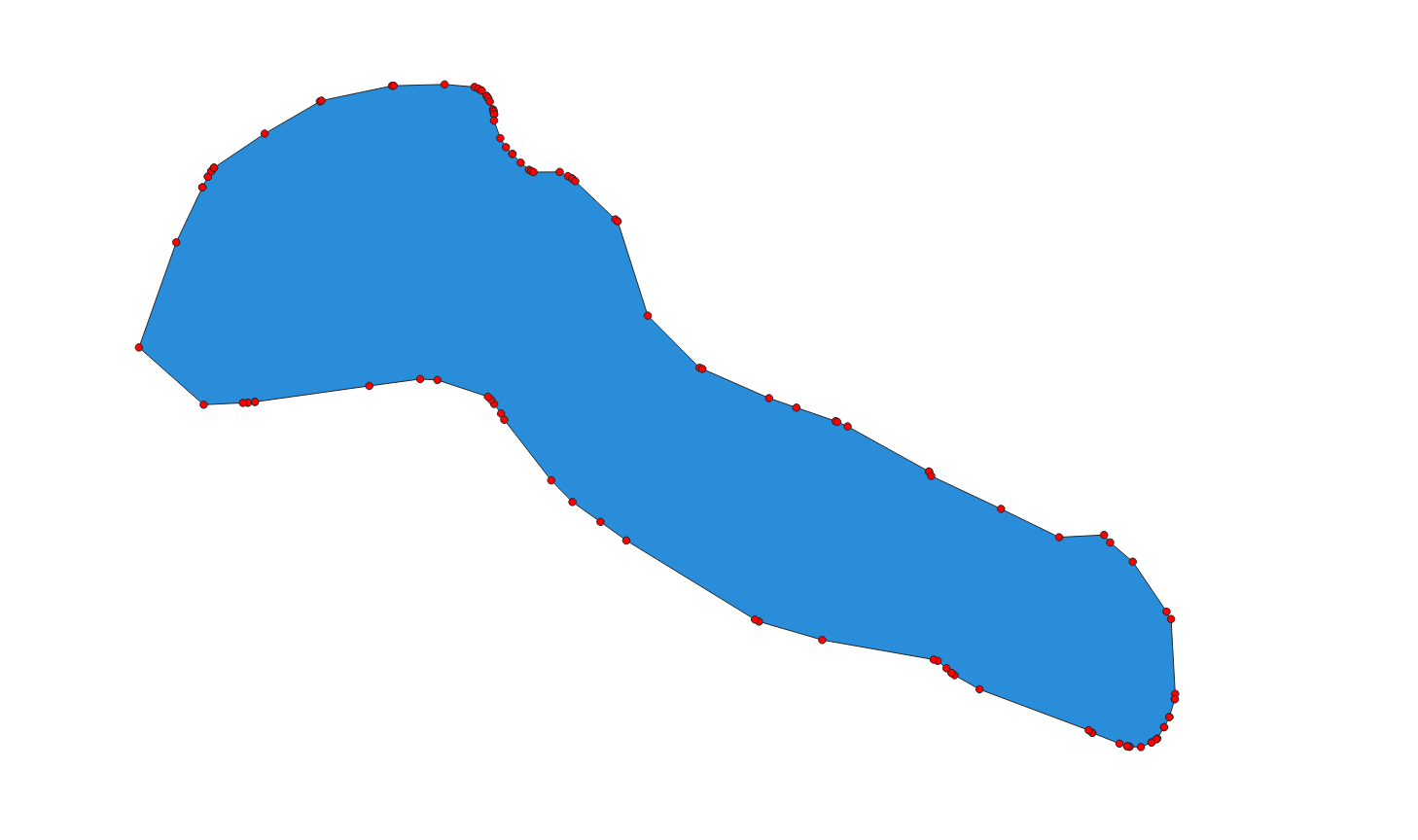I’m open to suggestions for a better way to do this (preferably with out needing to visit every vertex).

The next step is to move the code to an unsupported GeoTools module (probably `gt-process-geometry`) that will let people experiment with the code and add improvements as needed.